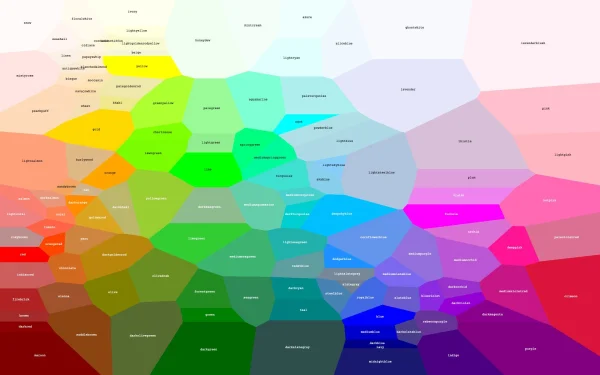Hmmmmmmm… 上圖是理想中的Voronoi Diagram

• 11/14 初測(基本功能+3點VD)：19.5 / 20
• 12/05 完測(完整功能+4點以上VD)：34 / 60
• 12/13 報告撰寫：?? / 20

commits數量應該不難看出花了不少時間在上面，但效果顯然很糟• import: 讀檔格式如下
• random: 隨機生成N個點，預設為6點可重複疊加
• save: 將point, edge存成import格式
• next case: 當讀入的檔有多筆case，依此按鈕進行下一筆testcase
• step by step: 依Divide-and-Conquer逐步執行
• run VD: 畫出Voronoi Diagram結果
• clear：清除畫布
• convex hull: 將所有的Convex Hull畫出，算是拿來dubug用的XD

• Operating System
• Python3.6 (Virtual environment)
• Package version
• imutils==0.5.3
• Kivy==1.11.1
• numpy==1.13.3
• scipy==0.19.1
• pyinstaller==3.5 (WindowsOS)

### Data Structure

• Point:
用Python的tuple資料型態以$(x, y)$儲存讀入的points，再放入list當作該testcase的point list。
• Edge
從Python環境而言，必須用兩點才能畫成一線，所以在資料結構上以$[(x1, y1), (x2, y2)]$方式儲存。另外一種是進行中垂線繪製時，由於除了該中垂線以原本$[(x3, y3), (x4, y4)]$ 儲存外，還必須記錄該線是以哪一條線作為中線基準，因此儲存方式為$[(x3, y3), (x4, y4), (form_x1, form_y1), (form_x2, form_y2)]$，方便後續進行VD。
• Convex Hull (CH)
前置作業為針對$x$座標將point list進行排序，因此CH function的回傳值為CH的描點順序。
• Voronoi Diagram (VD)
VD的資料型態為edge list，其中不乏上述Edge data structure的兩種儲存方式。

### Algorithm

• Convex Hull (CH)
利用排序先找到最外圍的任一point作為起點，窮舉平面上所有的點，利用向量積(cross product)判斷是否在圈內or圈外，順著外圍繞一圈。

• Voronoi Diagram (VD)
3點以內利用外心以及各邊的中點，考慮各種情況下畫出VD。
多點情況，利用Divide-and-Conquer進行實作，在遞迴至3點以下時將會直接回傳結果，此外將point set拆成兩邊進行遞迴後再合併，詳見如下(n為points count)。
f(point\ set)=\left{ \begin{aligned} Do\ nothing && n = 1 \ Return\ Line && n = 2 \ Return\ VD && n = 3 \ Return\ Merge(f(point\ set[0, n/2]),f(point\ set[n/2, n])) && n > 3 \ \end{aligned} \right.
針對Merge function，其步驟如下:

• step 0. Hyperplane預設在畫布由上往下描出。

• step 1. 將兩邊points set各自以y座標進行排序，找出最上面兩點作為參考點。

• step 2. 利用參考點畫出的中垂線作為Hyperplane，並且和兩邊VD的所有edge set找出最上面的交點，並記錄撞到的edge。

• step 3. 根據撞到的edge進行參考點移動，並消除多餘的線。

• step 4. 重複step 2和step 3，直到兩邊point set走到底為止。

(下面兩張圖各6點。藍線為CH; 綠線為VD; 紅線為Hyperplane)### Result